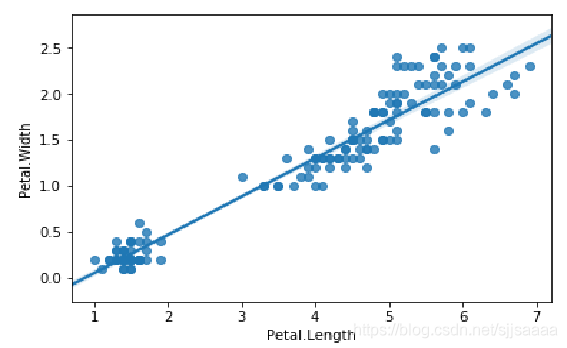python数据分析与可视化——利用Seaborn进行绘图黎明之道286 0 0

## 利用Seaborn进行绘图

Matplotlib绘图基本模仿MATLAB绘图库，其绘图风格和MATLAB类似。由于MATLAB绘图风格偏古典，因此，Python开源社区开发了Seaborn绘图模块，对Matplotlib进行封装，绘图效果更符合现代人的审美。
Seaborn属于Matplotlib的一个高级接口，使得作图更加容易。在多数情况下使用Seaborn能做出很具吸引力的图，而使用Matplotlib可以制作具有更多特色的图。应该把Seaborn视为iris.csvMatplotlib的补充，而不是替代物。

import seaborn as sns

Seaborn通过set函数实现风格设置。
seaborn.set(context=‘notebook’, style=‘darkgrid’, palette=‘deep’, font=‘sans-serif’, font_scale=1, color_codes=True, rc=None)

### 利用seaborn.set进行风格设置

import seaborn as sns
import numpy as np
import matplotlib as mpl
import matplotlib.pyplot as plt
def sinplot(flip=2):
x = np.linspace(0,20, 50)
for i in range(1,5):
plt.plot(x, np.cos(x + i * 0.8) * (9 - 2*i) * flip)
sinplot()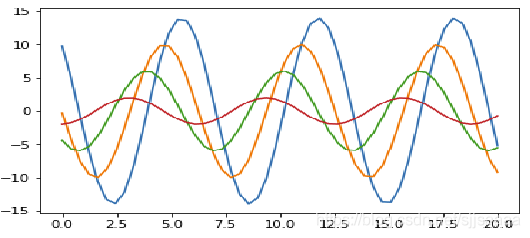sns.set(style='darkgrid',font_scale=1.5)
sinplot()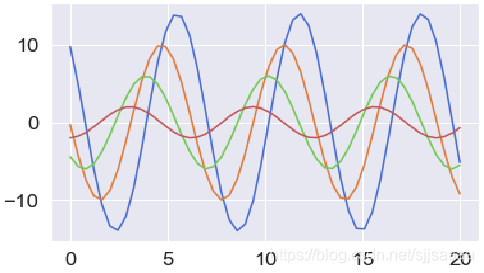sns.set()
sinplot() 

Seaborn有五个预设好的主题： darkgrid， whitegrid，dark，white，和 ticks，默认为darkgrid。

sns.set_style("whitegrid")
sinplot()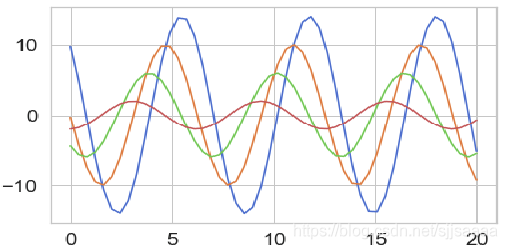Seaborn将matplotlib的参数划分为两个独立的组合。第一组是设置绘图的外观风格的，第二组主要将绘图的各种元素按比例缩放的，以至可以嵌入到不同的背景环境中。控制这些参数的接口主要有两对方法：

sns.set_style("whitegrid")
sinplot()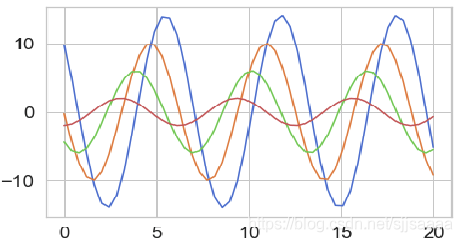sinplot()
sns.despine()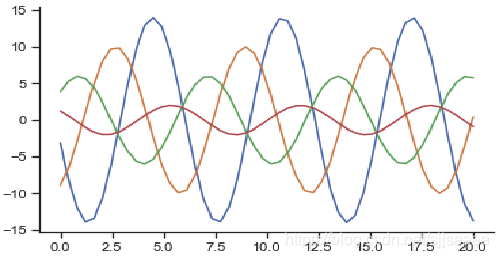despine（）方法中可以利用offset参数讲轴线进行偏置，另外，当刻度没有完全覆盖整个坐标轴的的范围时，利用trim参数限制已有坐标轴的范围。

sinplot()
sns.despine(offset=20, trim=True)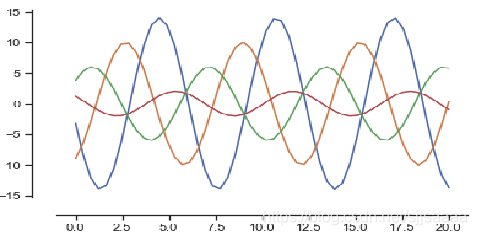sinplot()
sns.despine(left=True)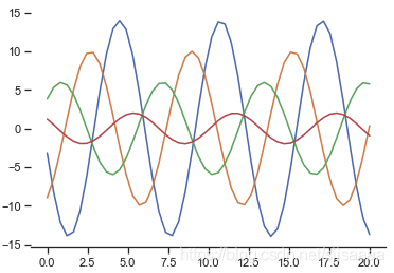sinplot()
sns.set(style='whitegrid',palette='muted',color_codes=True)
sns.despine(left=True,bottom=True)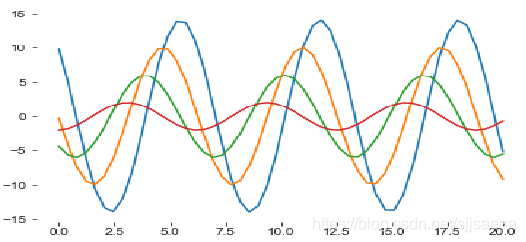with sns.axes_style("darkgrid"):
plt.subplot(2,1,1)
sinplot( )
plt.subplot(2,1, 2)
sinplot(-1)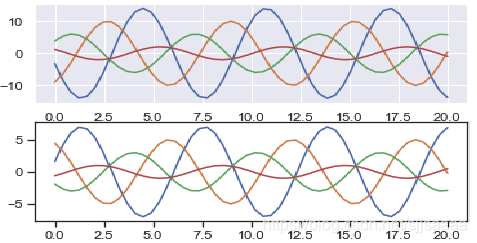seaborn中通过set_context()设置缩放参数，预设的参数有paper, notebook, talk, poster。默认为notebook。

sns.set_style("darkgrid", {"axes.facecolor": ".9"})
sinplot()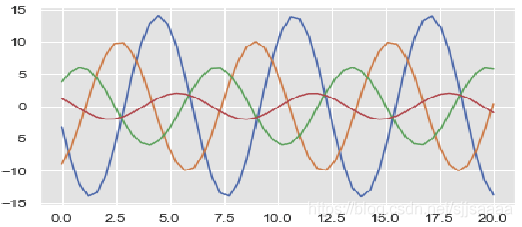sns.set_context("paper")
sinplot()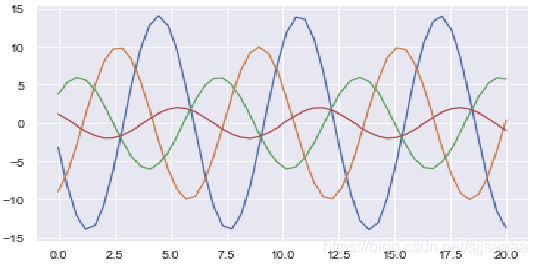sns.set_context("notebook", font_scale=1.8, rc={"lines.linewidth": 1.5})
sinplot()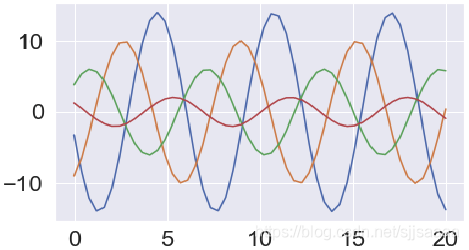### 常用的绘图

#### 直方图和密度曲线图

Seaborn中利用distplot( )和 kdeplot( )绘制直方图和密度曲线图，distplot( )为hist加强版，默认情况下绘制一个直方图，并嵌套一个对应的密度图。

import matplotlib.pyplot as plt
import seaborn as sns
import numpy as np
sns.set(palette="muted",color_codes=True)
rs=np.random.RandomState(10)
d=rs.normal(size=100)
f,axes=plt.subplots(2, 2, figsize=(7, 7), sharex=True)
sns.distplot(d, kde=False, color="b", ax=axes[0,0])
sns.distplot(d, hist=False, rug=True, color="r", ax=axes[0,1])
sns.distplot(d, hist=False,color="g", kde_kws={"shade":True}, ax=axes[1,0])
sns.distplot(d, color="m", ax=axes[1,1])
plt.show()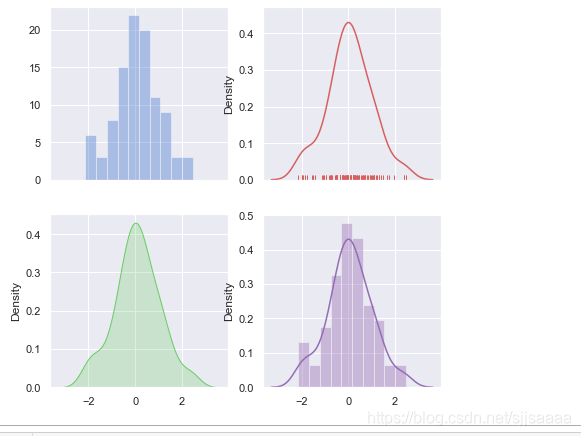#### 散点图

sns.set(style='white',color_codes=True) #设置样式
sns.stripplot(x=df_iris['Species'],y= df_iris['Petal.Width'],data=df_iris)
sns.despine()   #去坐标轴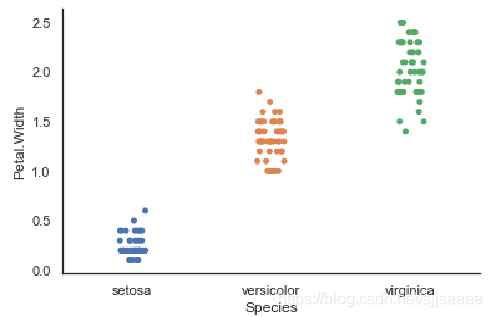sns.swarmplot(x=df_iris['Species'],y= df_iris['Petal.Width'],data=df_iris)
sns.despine()   #去坐标轴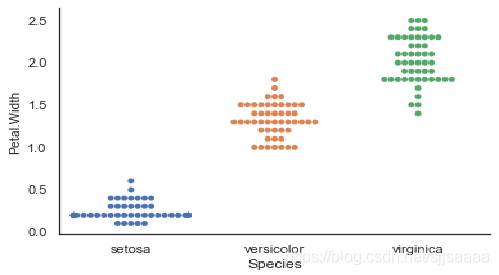#### 箱型图

df_iris=pd.read_csv('D:\dataset\iris.csv')
sns.boxplot(x=df_iris['Species'],y = df_iris['Petal.Width'])
plt.show()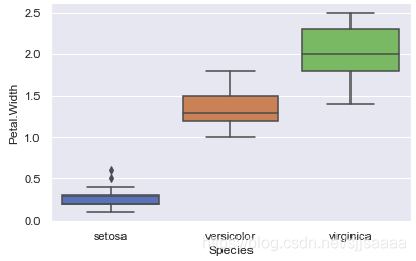df_iris=pd.read_csv('D:\dataset\iris.csv')
sns.set(style="ticks")
sns.boxplot(x=df_iris['Species'],y = df_iris['Petal.Width'])
plt.show()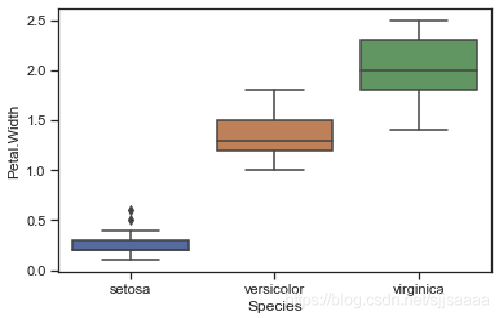#### pairplot

seaborn.pairplot(data, hue=None, hue_order=None, palette=None, vars=None, x_vars=None, y_vars=None, kind='scatter', diag_kind='auto', markers=None, height=2.5, aspect=1, dropna=True, plot_kws=None, diag_kws=None, grid_kws=None, size=None) 

pairplot主要展现的是变量两两之间的关系（线性或非线性，有无较为明显的相关关系）

df_iris=pd.read_csv('D:\dataset\iris.csv')
sns.set(style="ticks")
g = sns.pairplot(df_iris,vars=['Sepal.Length', 'Petal.Length'])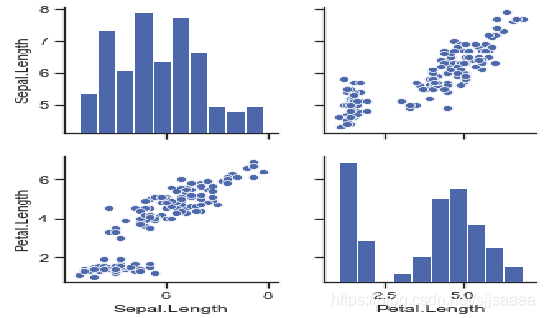#### 小提琴图

sns.set_style("whitegrid")
df_iris=pd.read_csv('D:\dataset\iris.csv')
ax = sns.violinplot(x=df_iris['Petal.Length'])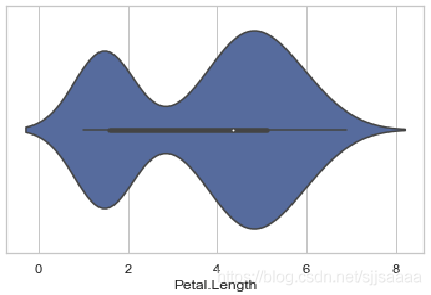#### 柱状图

df_iris=pd.read_csv('D:\dataset\iris.csv')
sns.barplot(x=df_iris['Species'],y=df_iris['Petal.Length'],data=df_iris)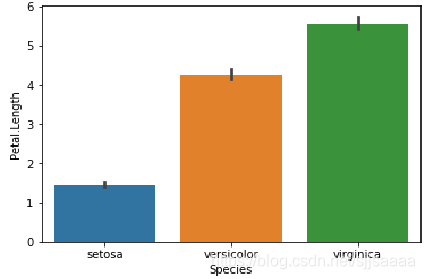sns.countplot(x='Species',data=df_iris)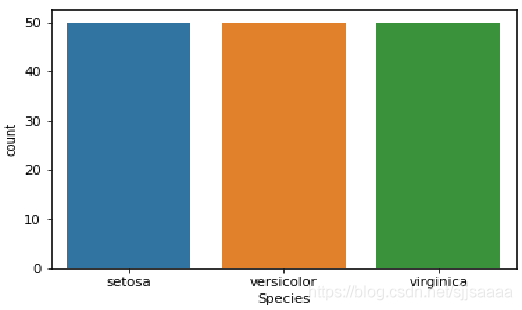#### 多变量图

sns.jointplot(x='Petal.Length',y='Petal.Width' ,data=df_iris)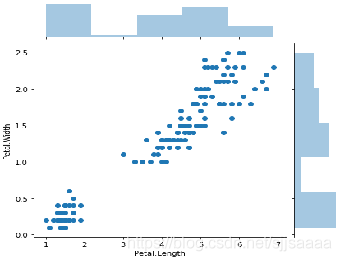ointplot(x='Petal.Length',y='Petal.Width' ,data=df_iris,kind='kde')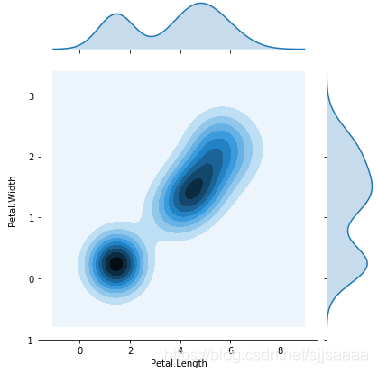#### 回归图

sns.regplot(x='Petal.Length',y='Petal.Width' ,data=df_iris)